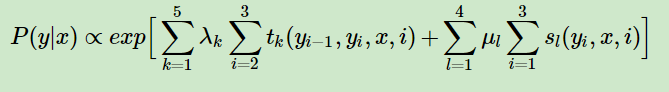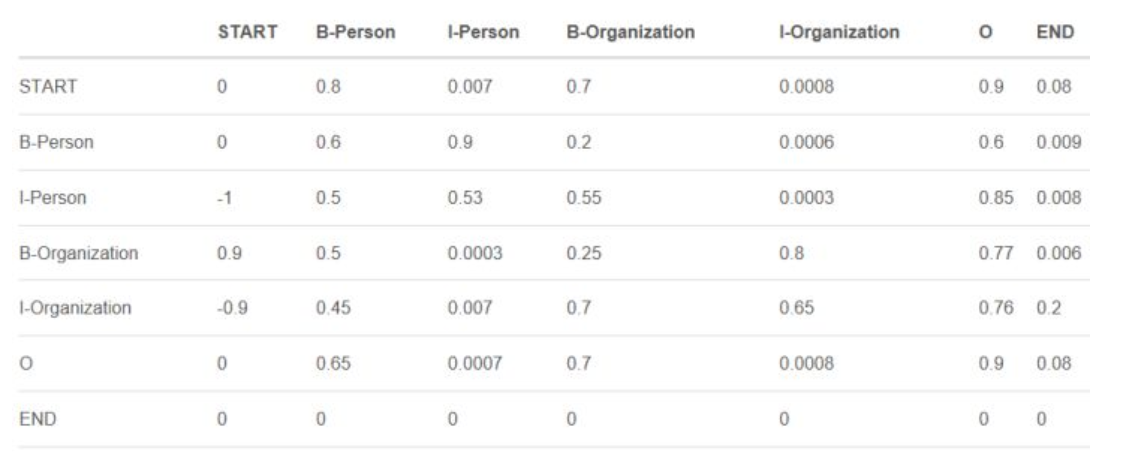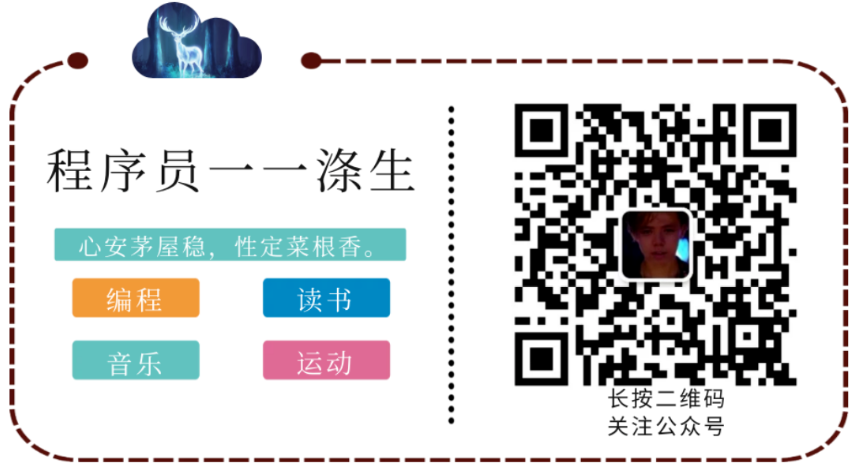Categories

# Learning to do with the depth of the named entity recognition (seven) -CRF Introduction

Remember introduced before the NER series of articles you can extract from the sentence out names, addresses, companies and other entities field, was only briefly mentioned the BERT + CRF model, BERT has been introduced in the last article before, This article will do a basic introduction to CRF. This article does not involve complex mathematical formulas obscure as much as possible, the purpose is just a quick overview of the basic concepts of CRF and its role in the field of natural language processing of named entity recognition.

What is CRF?

CRF, the full name of Conditional Random Fields, Chinese name: CRFs. Is a set of conditions for a given input sequence, another set of conditional probability distribution model of the output sequence.

When you can use CRF?

When the state of each position of the output sequence, to consider a state when adjacent positions. Here are two examples:
1, assuming that there are a bunch Xiaoming daily life photos, possible states are eating, bathing, brushing teeth, etc., in most cases, we are able to identify Xiaoming state, but if you see a Zhang Xiaoming photos toothy, in the absence of in the case of Xiao Ming neighboring state of conditions, it is difficult to judge that he was eating or brushing. In this case, you can use crf.
2, assuming that there is a word, this assumption is English, we have to determine the part of speech of each word, then the word for some, if not knowing the adjacent parts of speech of the word, it is difficult to accurately determine the part of speech of each word of. At this time, you can also use crf.

What is random?

Our first is what is random.
The collection of random variables is called a stochastic process.A stochastic process that is indexed by a spatial variable is called a random field.
A collection of random variables called a random process. A space by a random process variable index, called random.
In other words, a set of random variables according to a certain probability distribution of random assignment to a set position on a space, which gives the location of the random variable is a random field. For example, in the above example, Xiao Ming series of photographs are what make up a group of state position, we {eating, bathing, brushing} from a set of random variables values, random variables follow a probability distribution, a randomly assigned after a certain output position group of photos, and complete all the output position this group of photos of the state of the assignment, and location of these states called all over the airport.

Why is it called conditional random?

Answer this question you need to look at what is Markov random field, a position only if the assignment and the value of its adjacent location, but not with non-adjacent location and its value, then this is random a Markov random field. The assumptions used in the examples of speech tagging and Bob are in the right, because we can determine the current Luya photos by before a picture is brush your teeth or eat, according to the speech in front of a word can determine whether the current word speech grammatically.
Markov model is to generate observable state (words) based on implicit variables (POS), and CRFs is (word) discrimination implicit variables (POS) based on observation status. Or CRFs are given observation state (input sequence) set a priori conditions MRF. These are a priori conditions of the airport given Xiaoming photos, given English sentence, in the above example.

Mathematical description of CRF

Provided X and Y are random variables, P (Y | X) is a conditional probability distribution of X given Y when, if the random variable Y is composed of a MRF is said conditional probability distribution P (Y | X) is conditional random. In practical applications, such as the above two examples, we generally require X and Y have the same structure, as follows:
X = (X1, X2, … Xn), Y = (Y1, Y2, … Yn)
Such as speech tagging, we require that each word in the sentence speech input and output sequence is one to one.
X and Y have the same configuration CRF constitutes a linear chain Conditional Random Fields (Linear chain Conditional Random Fields, referred to as linear-CRF).
The above example is not mentioned in named entity recognition, but in fact named entity recognition principles and examples of the above is the same, but also uses a linear-CRF, it will be mentioned later.

How to extract the CRF feature?

There are two types CRF characteristic function is the state transition characteristics and features, characterized by the current node state (a state of output of a position of possible states is called a node) represents the state of the score, a node forwarding feature to spend to the current node metastasis scores expressed. Which loss function defined as follows:

CRF loss calculation function, need to use real path score (including the status points and transfer points), other scores all possible paths (including the transfer of state scores and scores). Path of the wording the word here is exemplified sequences corresponding to parts of speech, the real path represents the true speech sequences, other possible paths showing another part of speech sequence.
Here is the probability that the score before softmax, otherwise known as the probability of not standardized. Softmax effect is converted into a set of numerical values ​​between a group of 0-1, and is one of these values, so that a probability can be expressed.l shows a state wherein the definition of a word number, k is the number of transfer characteristics, i denotes the position of the word in the sentence.

sl and tk are respectively the transfer function characteristic function and status features.

and λk are respectively μl state transfer characteristic function and weight characteristic function weighting coefficients, the maximum likelihood estimation can be obtained.

Transition state scores and scores mentioned above are non-normalized log probability, the probability calculation is an addition, where exp is coupled to a logarithmic probability to normal probability. Will be divided by the actual calculation of a normalization factor Z (x), is actually a softmax process.

In the case only of CRF, wherein said Class 2 above functions are set manually good. Popular to say that the artificial set of characteristics observed sequence.
State artificially set template features, such as setting “a word is a noun,” and so on.
Artificially set template transfer characteristics, such as setting “a word is a noun, the adjective is a word” and so on.
When given a word, it calculates a feature score of this sentence according to the above-set feature templates, calculation of the time, if this sentence in line with characteristic features of template rules, the rules on the value of the feature 1, otherwise it is zero.
Entity Recognition performance is good or bad depends on two kinds of template feature set.
So if we can use the depth of the neural network the way, you can learn the characteristics obtained by the model himself, this is the reason for using BERT + CRF’s.

BERT named entity recognition and CRF is how fit?

B-Person I-Person O B-Location I-Location O B-Location I-Location I-Location O

That BERT layer each character learned a sentence most likely corresponds to the entity label is what this process is taken into account contextual information for each character left and right, but the maximum score corresponding to the output still marked entity may be incorrect not 100% correct, appears directly behind B followed by B, the probability of occurrence of the situation which marked the beginning of an I, it’s all possible, to reduce these problems is clearly incompatible rules, we can further improve the model predictions BERT accuracy. At this point some people think of using CRF to solve this problem.

CRF algorithm involves two kinds of characteristic functions, a feature is a function of the state, state score calculation, a transfer characteristic function is calculated metastasis score. The former can only be converted for the character at the current position of the entity to which marked the latter concern is the character of the current position and its location adjacent to the combination of which entities may have marked. BERT layers have fractional output state to the CRF layer, the CRF needs to learn a transfer layer further score matrix, which represents all the combinations between the state labels, such as we have here, B-Person I-Person B-Location I- Location O total of five states, sometimes the beginning and end of each plus a sTART and eND marked sentence, indicate the beginning and end of a sentence, then the time is seven kinds of state, then the two states (including their own and between their combination) had 7 * 7 = 49 kinds of the above said transition element is the matrix of scores of the 49 combinations of the fraction (or referred to as non-normalized probability), indicates the possibility of a combination of each. This matrix is ​​initially random initialization, after training by slowly will know which combination is more in line with the rules, which do not conform to the rules better. Thus bringing similar to the following constraints for the prediction model:

Beginning of the sentence should be “B-” or “O”, rather than “I-“.

“B-label1 I-label2 I-label3 …”, in this mode, it should be 1,2,3 category same category entity. For example, “B-Person I-Person” is correct, while “B-Person I-Organization” is wrong.

“O I-label” is wrong, named after the beginning of the entity should be “B-” rather than “I-“.Why can not manually mark to determine the rules of good writing and correction logic of it?
Because although artificial can determine predict whether the relationship before and after the labeling rules, but not know how to forecast does not comply with the rules to be adjusted, for example, we know that at the beginning of the sentence should be “B-” or “O”, rather than “I- “but what is the B- or O it? And for the next scene marked state very much, logic and artificial write workload is very large and complex.

CRF loss calculation function, need to use real path score (including the status points and transfer points), other scores all possible paths (including the transfer of state scores and scores). Wherein the state of the real path score is calculated based on the output of the trained model BERT, metastasis score is supplied from the transfer matrix of scores obtained CRF layer. State scores and scores of other transfer path is actually calculated in this way.

Finally, we calculate the true path of the principle point of view, the fact is the use of the Viterbi algorithm.

Viterbi algorithm

Concept: The two layers adjacent to the composition of the final optimum path from the optimum path to the end of the initial layer layers. The optimal path is the path to all nodes from each layer reaches a node in the current layer, or the maximum probability (, specifically self-defined or other reasons) the shortest distance route.
The core idea: one from each node on the path to reach the probability of a node of the current maximum level, the node must be in the maximum path. Because when the subsequent recalculation of the state transition probability that a state of a node to the next node (or a target node) is greater than a certain probability of other node to the next node in a certain state, as a target node to which will only depending on the current node to the destination node metastasis score and the target node status score, but regardless of which node to the current node on the optimal path of this judgment is beyond doubt. As shown below:

H, F between the transition probability is fixed, comprising H-> H and F-> F, are transferred from the matrix of scores, such as the FIG 0.6 0.084 * 0.3 * H0.084 blue box from a node, the node is a node on the path of maximum probability calculated on a layer, you might think, if this layer probability H-> F ratio is calculated F-> F small probability of how to do, is not on the Viterbi algorithm not the global optimal solution? In fact, this one to calculate the probability H-> F ratio F-> F small probability will not happen, We look at this layer (blue box) two state transition probabilities:

• H->F 0.084 * 0.3 * 0.6
• F-> F 0.027 * 0.6 * 0.6
Since the last number is the last layer to the state of node F fraction, so it is 0.6, then when the comparison may not be considered, thereby obtaining the following equation is calculated:

0.3 0.084 * The first number is the one obtained score, the score is the maximum of all paths, the maximum value is calculated: 0.084 * 0.3 * 0.3 = 0.3

0.6 0.027 * The first number is the one obtained score, the score is calculated: 0.027 * 0.6 * 0.3 = 0.04
According to the above logic, to obtain the following equation:

• 0.084 * 0.3 = 0.3 * 0.3 * 0.3 * 0.3
• 0.027 * 0.6 = 0.04 * 0.6 * 0.3 * 0.6

Here the first item 0.3 and 0.04, respectively, represent the state of fractions F and H on node layer, and we were set to a B, now known a * 0.3 * 0.3 = 0.084, b * 0.6 * 0.3 = 0.027, 0.084> 0.027, it is transferred back again, i.e. 0.3 * 0.084 0.027 *, must be greater than 0.6, if there are more layers, then a back calculation is multiplied by 0.3, it is multiplied by 0.6, of course, because 0.3 is transferred to a transition probability F H and F is 0.6 F, transferred to a transition probability, the transition probability is fixed.

After the highest score so the current state of the node layer, must be in the optimal path to the last layer in order recursion, in turn, each retrospective recorded maximum probability nodes, can be obtained the one optimum path.
FIG optimal path are: Start-H-H-F

to sum up

Named entity recognition, BERT responsible for learning rules and symbol to each word label corresponding physical input sentence, and CRF entity responsible for the transfer rules between adjacent label study.

ok,本篇就这么多内容啦~，感谢阅读O(∩_∩)O。